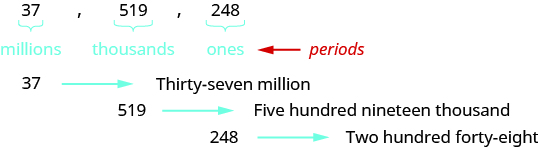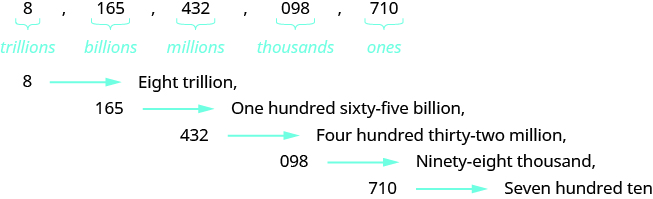## Writing Whole Numbers with Words

### Learning Outcomes

• Write a whole number given as digits in words
• Write a whole number given in words as digits

## Use Place Value to Name Whole Numbers

When you write a check, you write out the number in words as well as in digits. To write a number in words, write the number in each period followed by the name of the period without the ‘s’ at the end. Start with the digit at the left, which has the largest place value. The commas separate the periods, so wherever there is a comma in the number, write a comma between the words. The ones period, which has the smallest place value, is not named.So the number $37,519,248$ is written thirty-seven million, five hundred nineteen thousand, two hundred forty-eight.
Notice that the word and is not used when naming a whole number.

### Name a whole number in words

1. Starting at the digit on the left, name the number in each period, followed by the period name. Do not include the period name for the ones.
2. Use commas in the number to separate the periods.

### example

Name the number $8,165,432,098,710$ in words.

Solution

 Begin with the leftmost digit, which is$8$. It is in the trillions place. eight trillion The next period to the right is billions. one hundred sixty-five billion The next period to the right is millions. four hundred thirty-two million The next period to the right is thousands. ninety-eight thousand The rightmost period shows the ones. seven hundred tenPutting all of the words together, we write $8,165,432,098,710$ as eight trillion, one hundred sixty-five billion, four hundred thirty-two million, ninety-eight thousand, seven hundred ten.

### example

A student conducted research and found that the number of mobile phone users in the United States during one month in $2014$ was $327,577,529$. Name that number in words.

## Use Place Value to Write Whole Numbers

We will now reverse the process and write a number given in words as digits.

### Use place value to write a whole number

1. Identify the words that indicate periods. (Remember the ones period is never named.)
2. Draw three blanks to indicate the number of places needed in each period. Separate the periods by commas.
3. Name the number in each period and place the digits in the correct place value position.

### example

Write the following numbers using digits.

1. fifty-three million, four hundred one thousand, seven hundred forty-two
2. nine billion, two hundred forty-six million, seventy-three thousand, one hundred eighty-nine

### example

A state budget was about $\text{\77}$ billion. Write the budget in standard form.

### try it

Write each number in standard form:

1. The closest distance from Earth to Mars is about $34$ million miles.
2. The total weight of an aircraft carrier is $204$ million pounds.

The video below shows more examples of how to use place value to write the name of a whole number.